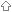Last update July 18, 2009

# Lexical Closures

Lexical closures basically amount to functions which remember their surrounding environment.

They differ from the DynamicClosures? of D in that they can remember this information even after the scope in which they were declared has terminated.Closure using a class objectClosure using templateClosure using CurryD2 adds support for closures

Lexical closures make lots of interesting things possible: Note: this won't work in D1.x. It's for illustration purposes only.```alias int delegate(int x) AddFunc; AddFunc addN(int n) { int add(int i) { return i + n; } return &add; // the add function captured will remember the value of n } void apply(int[] array, AddFunc func) { foreach (inout int i; array) { i = func(i); } } int main() { int[] numbers = ...; apply(numbers, addN(40)); // add 40 to each number }```

While the above example does demonstrate a limitation with using the stack frame for closure, there are several ways to implement effectively the same thing using different methods.

###Closure using a class object```alias int delegate(int x) AddFunc; AddFunc addN(int n) { class A { int n; this(int n) { this.n = n; } int add(int i) { return i + n; } } A a = new A(n); return &a.add; // the add function captured will remember the value of n } void apply(int[] array, AddFunc func) { foreach (inout int i; array) { i = func(i); } } void main() { int[] numbers = [1,2,3]; apply(numbers, addN(40)); // add 40 to each number }```

An example of two closure instance:```import tango.io.Stdout; // Safe but ugly. int delegate() countdown(int from) { class Log { int counter; this (int counter) {this.counter = counter;} int sub () {return counter--;} } return &(new Log(from)).sub; } void main () { int delegate () dg = countdown(10); Stdout.format ("dg:{}\n", dg()); // 10 Stdout.format ("dg:{}\n", dg()); // 9 Stdout.format ("dg:{}\n", dg()); // 8 Stdout.format ("dg:{}\n", dg()); // 7 int delegate () dg2 = countdown(10); Stdout.format ("dg2:{}\n", dg2()); // 10 Stdout.format ("dg2:{}\n", dg2()); // 9 Stdout.format ("dg:{}\n", dg()); // 6 }```

###Closure using template```alias int delegate(int n) AddFunc; template addN(int N) { AddFunc addN() { return delegate int (int i) { return i + N; }; } } void apply (int[] array, AddFunc func) { foreach (inout i; array) { i = func(i); } } void main () { int[] numbers = [1,2,3]; apply(numbers, addN!(40)); }```

PS. This is not really LexicalClosures(real closures), it may cause problem if you have 2 instance of template with same parameter. The following code shows this problem.```import tango.io.Stdout; int delegate () countdownT (int from) () { static counter = from; //Work both on gdc 0.24 w/ Linux & DMD 1.021 on Win int sub () {return counter--;} return ⊂ } int main () { int delegate () dg = countdownT!(10); Stdout.format ("dg:{}\n", dg()); // Should be 10 Stdout.format ("dg:{}\n", dg()); // Should be 9 Stdout.format ("dg:{}\n", dg()); // Should be 8 Stdout.format ("dg:{}\n", dg()); // Should be 7 int delegate () dg2 = countdownT!(10); Stdout.format ("dg2:{}\n", dg2()); //! ERROR, Should be 10, but 6 Stdout.format ("dg2:{}\n", dg2()); //! ERROR, Should be 9, but 5 Stdout.format ("dg:{}\n", dg()); //! ERROR, Should be 8, but 4 return 0; }```

###Closure using Curry```alias int delegate(int x) AddFunc; AddFunc addN(int n) { int add(int a, int b) { return a + b; } return Curry(&add, n); // the add function captured will remember the value of n } void apply(int[] array, AddFunc func) { foreach (inout int i; array) { i = func(i); } } void main() { int[] numbers = [1,2,3]; apply(numbers, addN(40)); // add 40 to each number } /* R is return type * A is first argument type * U is TypeTuple of rest of argument types */ R delegate(U) Curry(R, A, U...)(R delegate(A, U) dg, A arg) { struct Closure { typeof(dg) originalDelegate; A value; R subDelegate(U u) { return originalDelegate(value, u); } } Closure* f = new Closure; f.originalDelegate = dg; f.value = arg; return &f.subDelegate; }```

###D2 adds support for closuresFull closure support was added to D 2.007, the scope parameter storage class means the parameter will not 'escape' the scope of the function invocation. Using this on delegate parameters will prevent some closure allocations by the calling function.

The following code gives the correct output.```import std.stdio : writeln; alias int delegate(int x) AddFunc; AddFunc addN(int n) { int add(int i) { return i + n; } return &add; // the add function captured will remember the value of n } void apply(int[] array, /* scope */ AddFunc func) { foreach (inout i; array) { i = func(i); } } int main() { int[] numbers1 = [1,2,3,4,5,6]; int[] numbers2 = numbers1.dup; apply(numbers1, addN(40)); // add 40 to each number writeln(numbers1); apply(numbers2, addN(20)); // add 20 to each number writeln(numbers2); return 0; }```

Outputs:```[41 42 43 44 45 46] [21 22 23 24 25 26]```

| | | | | | | | | |

(date of last change: July 18, 2009 4:16 )